# Course Catalog

Math Olympiad Program – Designed by Evan Chen– One of the coaches of the USA IMO Team

– Co-Exam Coordinator for the USA Olympiad Team Selection Tests

– Member of the USA Math Olympiad (USAMO) Committee

Advanced Math Program – Foundations Level – Designed by Justin Stevens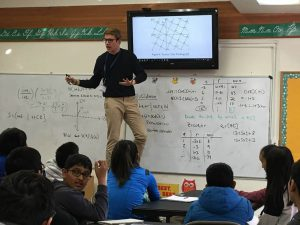“- Author of Olympiad Number Theory Through Challenging Problems Book

– “Our teacher, Justin, was a legend.” 2018 Camp Participant

– “Justin is a crazy good teacher and understands kids.” 2018 Camp Participant

– “Justin is the most awesome teacher EVER!!!’ 2018 Camp Participant”

Advanced Math Program – Mastery Level – Designed by Alex Toller“- Co-author of the book series Proofs in Competition Math,

– The highest scorer at the Nevada MathCounts State competition in Middle School,

– Studies mathematics at the University of California, Berkeley.

Courses to Choose From and Who Our Math Program is For

1- Students who would like to excel in school mathematics

Courses to choose from:

– Advanced Middle School Math with MathCounts/AMC 8-10 Problems

– Advanced High School Math with AMC 10/12 Problems

If you would like to get more information on AMC 8, 10, 12 and AIME competitions, please visit Mathematical Association of America’s American Mathematics Competitions (AMC) Page.

2- Students who would like to go above and beyond school mathematics

Courses to choose from:

– Proof Mathematics with AIME Problems

This course is a part of our Math Olympiad Program.

### Courses

Course Description:
The main objective of Algebra-1 is to provide the students with a strong background for all mathematical courses ahead. The basics of algebraic problem-solving are systematically taught. The students will gain a good grasp of the following topics: Basics of Algebra, equations, inequalities, functions at introductory level, linear functions, quadratic functions, equation and inequality systems, exponential functions, polynomials with factoring, radical expressions and equations, and data analysis and probability. Common Core State Standards will be taught and reinforced as the students learn how to practice these concepts in real-life situations. This course prepares students to take Honors Algebra-1, 2 and other upper level related courses.

Prerequisites:
Pre Algebra or 8th Grade Math, or placement test.

Common Core Standards Covered in this course:
NRN.1, NRN.2, NRN.3, NQ.1, NQ.2, NQ.3, NCN.1, NCN.2, NCN.3, NCN.4, NCN.5, NCN.6, NCN.7, NCN.8, NCN.9, ASSE.1, ASSE.1a, ASSE.1b, ASSE.2, ASSE.3, ASSE.3a, ASSE.3b, ASSE.3c, ASSE.4, AAPR.1, AAPR.2, AAPR.3, AAPR.4, AAPR.5, AAPR.6, AAPR.7, ACED.1, ACED.2, ACED.3, ACED.4, AREI.1, AREI.2, AREI.3, AREI.4, AREI.4a, AREI.4b, AREI.5, AREI.6, AREI.7, AREI.8, AREI.9, AREI.10, AREI.11, AREI.12, F.IF.1, F.IF.2, F.IF.3, F.IF.4, F.IF.5, F.IF.6, F.IF.7, F.IF.7a, F.IF.7b, F.IF.7c, F.IF.7d, F.IF.7e, F.IF.8, F.IF.8a, F.IF.8b, F.IF.9, F.BF.1, F.BF.1a, F.BF.1b, F.BF.1c, F.BF.2, F.BF.3, F.BF.4, F.BF.4a, F.BF.4b, F.BF.4c, F.BF.4d, F.BF.5, F.LE.1, F.LE.1a, F.LE.1b, F.LE.1c, F.LE.2, F.LE.3, F.LE.4, F.LE.5, S.ID.1, S.ID.2, S.ID.3, S.ID.4, S.ID.5, S.ID.6, S.ID.6a, S.ID.6b, S.ID.6c, S.ID.7, S.ID.8, S.ID.9

Textbook:

Mathematics Enhancement Programme

Course Outcomes:

After completing, students will be able to:

• Write expressions and evaluate them with unknown values,
• Use properties to simplify expressions,
• Identify and differentiate the types of relationships that can be represented by proportions.
• Use inverse operations to simplify and find equivalent equations,
• Solve equations using mathematical operations and equality properties,
• Write and plot inequalities,
• Model real-world situations using functions.
• Model and analyze real-world situations using a system of equations or inequalities,
• Simplify expressions by applying properties of exponents,
• Explore the characteristics of exponential functions,
• Explore polynomials through adding, subtracting, multiplying and factoring, and apply real number properties to manipulate polynomial expressions,
• Plot and identify characteristics of quadratic functions,
• Plot and simplify rational expressions,
• Figure out different strategies to find solutions for quadratic equations,
• Add, subtract, multiply and divide using radicals and learn how to rationalize the denominator of a radical expression,
• Multiply and divide rational expressions and divide polynomials,
• Interpret data in a real-world terms,
• Analyze data with multiple approaches.

Topics Covered In This Course:

• Review of Pre-Algebra
• Solving Equations
• Solving Inequalities
• Introduction to Functions
• Linear Functions
• Systems of Equations and Inequalities
• Polynomial and Factoring
• Rational Expressions and Functions
• Data Analysis and Probability

Course Description:

This course covers the required concepts of Euclidean geometry including definitions, postulates, and theorems. Throughout the course, Common Core standards are taught and reinforced as the student learns how to apply the concepts in real life situations. Areas of study include tools and language of geometry, reasoning and proof, parallel and perpendicular lines, congruent triangles, relationships within triangles, polygons and quadrilaterals, similarity, right triangles and trigonometry, transformations, area, surface area and volume, circles, and probability. This course prepares students to take Honors Geometry and other upper level related courses.

Prerequisites:

Algebra I or placement test.

The Next Generation Science Standards (NGSS) Codes:

G.CO.1, G.CO.2, G.CO.3, G.CO.4, G.CO.5, G.CO.6, G.CO.7, G.CO.8, G.CO.9, G.CO.10, G.CO.11, G.CO.12, G.CO.13, G.SRT.1, G.SRT.1a, G.SRT.1b, G.SRT.2,  G.SRT.3, G.SRT.4, G.SRT.5, G.SRT.6, G.SRT.7, G.SRT.8, G.SRT.9, G.SRT.10, G.SRT.11, G.C.1, G.C.2, G.C.3, G.C.4, G.C.5, G.GPE.1, G.GPE.2, G.GPE.3, G.GPE.4, G.GPE.5, G.GPE.6, G.GPE.7, G.GMD.1, G.GMD.2, G.GMD.3, G.GMD.4, G.MG.1, G.MG.2, G.MG.3

Textbook:

Mathematics Enhancement Programme

Course Outcomes:

Upon completion of this course, you should be able to:

• Understand and use the tools and language of Geometry through the exploration of points, lines, planes and angles.
• Understand, apply and use logical reasoning.
• Understand and apply the properties of parallel and perpendicular lines.
• Understand how special lines in triangles relate, and prove that two triangles are congruent.
• Understand and apply the properties of different types of polygons.
• Prove triangles are similar, and how to use the fact that two triangles are similar to find lengths of sides, and find out how the sides of a right triangle are related.
• Perform transformations and apply them to the real world.
• Use and apply area formulas, and finding the surface areas and volumes of three-dimensional figures.
• Apply theorems you learned in earlier chapters to segments touching circles.
• Use conditional probability, rules or probability, to use probability to make decisions

Topics Covered In This Course:

• The Tools and Language of Geometry
• Reasoning and Proof
• Parallel and Perpendicular Lines
• Congruent Triangles
• Relationships within Triangles
• Similarity
• Right Triangles and Trigonometry
• Transformations
• Area
• Surface Area and Volume
• Circles
• Probability

Course Description:

Algebra 2 is designed to build on algebraic and geometric concepts. Throughout the course, Common Core standards are taught and reinforced as the student learns how to apply the concepts in real-life situations. It develops advanced Algebra skills such as Algebra 2 review, function families, quadratic functions and complex numbers, polynomials expressions and equations, exponential and logarithmic functions, rational functions, statistics, periodic functions and trigonometry, and applying trigonometric functions. This course prepares students to take other upper level related courses.

Prerequisites:

Algebra 1 and Geometry, or placement test.

The Next Generation Science Standards (NGSS) Codes:

NRN.1, NRN.2, NQ.2, NCN.1, NCN.2, NCN.7, NCN.8, NCN.9, ASSE.1a, ASSE.2, ASSE.3a, ASSE.4, AAPR.1, AAPR.2, AAPR.3, AAPR.4, AAPR.5, AAPR.6, ACED.1, ACED.2, ACED.3, ACED.4, AREI.1, AREI.2, AREI.4, AREI.4a, AREI.4b, AREI.6, AREI.7, AREI.11, F.TF.1, F.TF.2, F.TF.5, F.TF.8, F.IF.1, F.IF.2, F.IF.3, F.IF.4, F.IF.5, F.IF.6, F.IF.7, F.IF.7b, F.IF.7c, F.IF.7e, F.IF.8, F.IF.9, F.BF.1, F.BF.1b, F.BF.3, F.BF.4, F.BF.4a, F.LE.4, S.ID.4, S.ID.6, S.IC.1, S.IC.2, S.IC.3, S.IC.4, S.IC.5, S.IC.6, S.MD.6, S.MD.7

Textbook:

Mathematics Enhancement Programme

Course Outcomes:

Upon completion of this course, you should be able to:

• Use algebraic expressions to represent patterns,
• Solve equations and inequalities,
• Solve absolute value equations,
• Use functions to model real world situations,
• Work with functions,
• Analyze transformations and characteristics of function families,
• Find the vertex and standard form of a quadratic equation,
• Gain an understanding of complex numbers,
• Classify, graph, and define end behavior of polynomial functions,
• Analyze the factored form of the polynomials and write polynomial functions from their zeros,
• Solve polynomial functions by graphing and factoring,
• Divide polynomials by long division and synthetic division,
• Model polynomial functions and identify the effect of transformations of polynomial functions,
• Work with radicals as a part of a function, equation, or by themselves,
• Add, subtract, multiply and divide functions,
• Find the composite of two functions,
• Find the inverse of a relation or a function,
• Understand the relationship between exponential and logarithmic functions and model exponential and logarithmic functions,
• Graph rational functions,
• Solve rational equations,
• Apply theoretical and experimental probabilities and compare data sets,
• Relate geometric measurements to trigonometry, to define radians, how to use radian measures and write and graph functions to describe periodic data,
• Verify trigonometric identity,
• Solve trigonometric equations and to solve real-world problems involving right triangles by using trigonometric ratios

Topics Covered In This Course:

• Review of Algebra 1 topics
• Quadratic Functions and Complex Numbers
• Polynomial Expressions and Equations
• Composite and Inverse Functions
• Exponential and Logarithmic Functions
• Rational Functions
• Statistics
• Periodic Functions and Trigonometry
• Applying Trigonometric Functions

Course Description:

The course is intended for students with a strong background in Trigonometry and Algebra.  It prepares students to take AP Calculus AB or a college calculus course. A range of topics including polynomial functions, rational functions, arithmetic and geometric progressions, trigonometric functions and their inverses, trigonometric identities, plots of trigonometric functions, combinations and permutation, theory of equations, polar coordinates, mathematical induction, and parametric equations is covered.

Prerequisites:

Algebra 1, 2 and Geometry, or better or equivalent by testing.

The Next Generation Science Standards (NGSS) Codes:

MP.1, MP.2, MP.3, MP.4, MP.5, MP.6, MP.7, MP.8, N-CN.1, N-CN.2, N-CN.3, N-CN.4, N-CN.5, N-CN.6, N-CN.7, N-CN.8, N-CN.9, N-VM.1, N-VM.2, N-VM.3, N-VM.4, N-VM.4a, N-VM.4b, N-VM.4c, N-VM.5, N-VM.5a, N-VM.5b, N-VM.6, N-VM.7, N-VM.8, N-VM.9, N-VM.10, N-VM.11, N-VM.12, A-REI.3, A-REI.4, A-REI.4a, A-REI.4b, A-REI.5, A-REI.6, A-REI.7, A-REI.8, A-REI.9, A-REI.10, A-REI.11, A-REI.12, A-APR.2, A-APR.3, A-APR.4, A-APR.5, A-APR.6, A-APR.7, F-IF.2, F-IF.3, F-IF.4, F-IF.5, F-IF.7, F-IF.7a, F-IF.7b, F-IF.7c, F-IF.7d, F-IF.7e, F-IF.8, F-IF.8a, F-BF.1, F-BF.1a, F-BF.1b, F-BF.1c, F-BF.2, F-BF.3, F-BF.4, F-BF.4a, F-BF.4b, F-BF.4c, F-BF.4d, F-TF.1, F-TF.2, F-TF.3, F-TF.4, F-TF.5, F-TF.6, F-TF.7, F-TF.8, F-TF.9, G-SRT.6, G-SRT.7, G-SRT.8, G-SRT.9, G-SRT.10, G-SRT.11, G-GPE.1, G-GPE.2, G-GPE.3

Textbook:

Mathematics Enhancement Programme

Course Outcomes:

After successful completion of this course, students should be able to:

• Determine derivatives of functions and explain the results with respect to their application.
• Calculate marginal cost, marginal revenue and marginal profit using derivatives.
• Calculate higher derivatives and interpret the results.
• Draw plots of polynomial functions.
• Determine domains and limits of rational functions and polynomials.
• Determine inflection points, critical points and relative extreme points, and present the results in various settings.
• Calculate average and total sales using results obtained through integration via substitution.
• Understand and apply the Generalized Power Rule and the Chain Rule.
• Conduct optimization of the continuous functions on closed intervals.
• Solve problems involving depreciation, interest, demand, revenue, advertising, decay and elasticity of demand using logarithmic and exponential functions.
• Integrate definite and indefinite exponential, logarithmic and polynomial functions and express the results in various application formats.
• Calculate derivative using implicit differentiation.
• Calculate the area between curves and the average value over an interval using integration.

Topics Covered In This Course:

• Functions and Graphs: Basic, Composite and Inverse functions and their graphs.
• Polynomial and Rational Functions
• Trigonometric Functions
• Analytic Trigonometry
• Additional Topics in Trigonometry: Polar Coordinates, Matrices, Complex Numbers in Polar Form (DeMoivre’s Theorem)
• Conic Sections and Analytic Geometry: The Ellipse, Hyperbola, Parabola, Parametric Equations
• Sequences, Induction, and Probability
• Introduction to Calculus

### Courses

Advanced Middle School Math with MathCounts/AMC 8-10 Problems

This course covers the main topics in middle school math. Students will be mastering these topics while solving challenging problems at the level of or from MathCounts, AMC-8, AMC 10 and similar competitions. Students go above and beyond Common Core standards in this brain-stimulating course. Students will also solve mathematical puzzles and cyphers and learn topics that are typically not covered at traditional school settings.

Recommended Grade Levels: Although we do not limit students by grade level, this course is typically recommended for students in grades 4th-8th.

Course Description: This course will familiarize students with the essential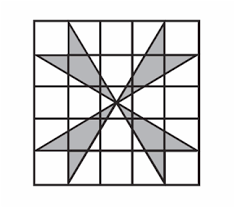concepts and techniques in Pre-Algebra, Algebra I, Geometry, Number Theory and Combinatorics. We will have a specific emphasis on problem solving where the students will constantly be challenged to think creatively.

Contest Preparation: MathCounts, AMC 8, AMC 10.

Course Objectives: As of the completion of this course, students will:

1. Have complete mastery of concepts covered in standard Pre-Algebra, Algebra I and Geometry courses, as well as topics not covered in traditional school curriculum.

2. Be able to explain and employ important theorems and techniques used in Combinatorics, Number Theory, and Geometry.

3. Be able to reduce unfamiliar problems to basic principles, and cleverly employ techniques they’ve learned to find shortcuts in solution methods.

Teaching Philosophy: We believe that building good problem-solving skills is as (if not more) important than knowing lots of theorems. As such, although the course will cover a considerable amount of material, the main emphasis will be on building problem-solving intuition and training students to think creatively when faced with classes of problems they’ve never seen before.

Class Participation: Students are expected to actively participate in class. We will employ the Socrates method, which is a cooperative dialogue between the students and teacher to stimulate critical thinking. Students will also collaborate with classmates while solving challenging problems.

Curriculum: The course curriculum is owned and copyright by CyberMath Academy. The class material is composed of unique blends of problems hand picked from prestigious competitions from around the globe, along with many historical problems and fascinating puzzles.

Information on Summer Camp Attendance Options (Academic Year Courses cover all topics)

Full-Day Program: Students who would like to master all topics should register for full-day program

Half-Day Program: Students who would like to master only Pre-Algebra and ALgebra-I topics alongside Number Theory can choose this option. Students can also choose to enroll only in morning classes.

Algebra

• Ratios and Proportions
• Algebraic Expressions
• Linear Equations
• Functions
• Inequalities
• Polynomial Expressions
• Pascal’s Triangle
• Binomial Theorem

Combinatorics

• Counting
• Statistics
• Probability
• Permutations
• Combinations

Number Theory

• Divisibility
• GCD and LCM
• Prime Factorization
• Modular Arithmetic
• Sequences and Series
• Gauss’s Formula

Geometry

• Angles
• Triangles
• Pythagorean Theorem
• Polygons
• Circles
• Perimeter, Area and Volume
• Coordinate Geometry
• 3D Geometry

Advanced Middle School Math with MathCounts/AMC 8-10 Problems – Mastery Level

Learning objectives

Upon completing this course, you will:

• Have complete mastery of concepts covered in standard Pre-Algebra, Algebra I and Geometry courses, as well as topics not covered in traditional school curriculum.
• Be able to explain and employ important theorems and techniques used in Combinatorics, Number Theory, and Geometry.
• Be able to reduce unfamiliar problems to basic principles, and cleverly employ techniques they’ve learned to find shortcuts in solution methods.

In this class in particular, I would like to emphasize concepts in mathematics that are more versatile and apply to other facets of your intellectual pursuits. The following is a more comprehensive list of goals that would serve you well in not just math courses, but the field of mathematics:

• Be able to construct geometric figures and diagrams from the ground up (rigorously, not using approximations or “it looks close enough”).
• Relate concepts and ideas to other areas of math, or even make inter-disciplinary connections.
• Associate specific queries and commands in problems with certain formulas and their applications.
• Re-derive generalizations of theorems, or entirely different theorems, from the principal ideas of a proof.
• Exercise the appropriate level of mathematical rigor in solutions, and especially in formal proofs. This entails the proper use of theorems, lemmas, and corollaries in a proof, and proving each step of a proof that, in any sense of the word, is not trivial. Essentially, you should be able to recognize the key differences between intuition (implicit knowledge) and mathematically rigorous proof.

Problem sets

Each problem set emphasizes at the forefront two particular key skills in mathematics: innovation in problem solving and the craft of mathematical writing. The goals of good mathematical writing are fundamentally similar to the goals of good English writing, in that a piece of mathematical prose should aim to convey a mathematical idea in as few words and as elegantly as possible. The point of a proof is to convince the reader of a truth; that you not only know what you’re talking about, but can convey your ideas in such a manner that your reader does not get lost in trying to figure out your argument, or have any doubts as to whether or not the logic behind your proof actually stands. We call these kinds of arguments rigorous; that is, there is no room to dispute them, on the grounds that they have covered all the necessary bases. A mathematical proof cannot be debatable as a matter of opinion; it must be set in stone-cold, logical thinking that builds off of axioms and known truths and makes no unfounded assumptions or guesses. You are Sherlock Holmes, and your mathematical toolbox is your Watson.

Essential topics

• Mathematical proof!
– What is a proof? And why is it important?
– Proof methods (Direct, contradiction, induction (strong, engineer’s), contrapositive, non-constructive,
others)
– Examples of false proofs
– Lots and lots of proof-writing practice!

• Algebra:
– Pre-algebra topics (review)
∗ The real number system
∗ Decimals, fractions, percentages, ratios
∗ Word problems
∗ Scientific notation
∗ Exponents, logarithms, and radical expressions
∗ Simplifying expressions (combining like terms)
∗ Factoring

– Algebra 1 topics (semi-review)
∗ What is a function?
∗ Inverse functions
∗ Systems of equations
∗ Basic inequalities
∗ Polynomials and their roots (+Vieta’s formulas)
∗ Rate problems

– Sequences and series
– Pascal’s triangle + binomial theorem
– Non-real numbers and the number hierarchy
– Substitutions

• Discrete math:
– Logic
∗ Propositional logic and rules
∗ Types of statements
∗ Statements vs. non-statements – Set theory
∗ Introduction to sets (terminology, definitions)
∗ Subsets
∗ Relations and functions
∗ Injections, surjections, bijections
∗ Cardinal and ordinal numbers
∗ All infinities are not created equal

– Number theory
∗ Properties of primes
∗ Studying the prime distribution
∗ Bases and modular arithmetic
∗ The interplay of GCD and LCM (and B ́ezout’s Theorem)
∗ Fermat’s Little Theorem, Wilson’s Theorem (and proofs)
∗ Euler’s (totient) theorem

– Combinatorics:
∗ Basic rules of counting
∗ Over/under-counting
∗ Inclusion-Exclusion Principle
∗ Pigeonhole principle
∗ Combinations, permutations
∗ Combinatorial identities (stars and bars, Pascal’s identity)
∗ Probability. Basic definitions/rules, conditional probability, applications.
∗ Expected value and linearity of expectation

– Statistics
∗ Introduction to terminology
∗ Statistical fallacies
∗ Interpreting data
· Different types of graphs
· Correlation vs. causation
· Standard deviation, variance, and data distributions

• Geometry:
– Fundamental definitions
– Axioms and postulates
– Triangle focus: congruence and similarity
– Coordinate geometry (and coordbashing tactics)
– 3D geometry (planes, cross-sections, volume formula proofs)
– Inscribed angle theorem
– Angle chasing
– Power of a point
– Overview of trigonometry:
∗ Definitions (sin, cos, tan and their reciprocals/inverses)
∗ Trig identities and their a priori derivations
∗ Applications to algebra (complex numbers, etc.)

– Laws of sines, cosines
– Polar coordinates and complex numbers

Supplementary topics
• Matrix algebra:
– Definitions
– Matrix arithmetic
– Elementary row/column operations – The determinant
– Introduction to vectors

• Advanced inequalities: AM-GM-HM-QM, Cauchy, etc.
• Some special geometric configurations.
• Graph theory (definitions, applications)
– Nodes, edges
– Identities
– Handshake theorem (combo)
– The Konigsberg bridge problem and its implications
– Trees/forests/binary trees
– Combinatorial and real-world applications

Special topics
• Extremely cursory computational intro to calculus (what’s a limit/derivative/integral, area under the curve, applications)
• Game theory (winstrats, prisoner’s dilemma)
• Cursory overview of Markov chains and decision processes (informally “states bashing”)
• Hilbert/Millennium/other open problems

Advanced High School Math with AMC 10/12 Problems

This course prepares students for American Mathematics Competitions 10 and 12 and the non-proof parts of AIME. The topics taught include the entire high school curriculum including trigonometry, advanced algebra, precalculus and advanced geometry, but exclude calculus. Our curriculum also includes some additional challenging and brain-stimulating topics outside of the traditional school curriculum.

Recommended Grade Levels: Although we do not limit students by grade level, this course is typically recommended for advanced 7th and 8th graders and high school students.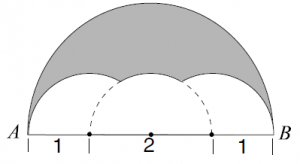Course Description: This course will familiarize students with the essential concepts and techniques in Algebra II, PreCalculus, Combinatorics, Number Theory, and Geometry. We will have a specific emphasis on problem solving where the students will constantly be challenged to think creatively.

Contest Preparation: AMC 10/12, AIME, ARML, Mandelbrot, Purple Comet.

Course Objectives: As of the completion of this course, students will:

1. Have complete mastery of concepts covered in standard Algebra II and PreCalculus courses, as well as more advanced topics (such as Vieta’s formulas, Complex Numbers, and manipulation of Series).

2. Be able to explain and employ important theorems and techniques used in Combinatorics, Number Theory, and Geometry.

3. Be able to reduce unfamiliar problems to basic principles, and cleverly employ techniques they’ve learned to find shortcuts in solution methods.

Teaching Philosophy: We believe that building good problem-solving skills is as (if not more) important than knowing lots of theorems. As such, although the course will cover a considerable amount of material, the main emphasis will be on building problem-solving intuition and training students to think creatively when faced with classes of problems they’ve never seen before.

Class Participation: Students are expected to actively participate in class. We will employ the Socrates method, which is a cooperative dialogue between the students and teacher to stimulate critical thinking. Students will also collaborate with classmates while solving challenging problems.

Curriculum: The course curriculum is owned and copyright by CyberMath Academy. The class material is composed of unique blends of problems hand picked from prestigious competitions from around the globe, along with many historical problems and fascinating puzzles.

Information on Summer Camp Attendance Options (Academic Year Courses cover all topics)

Full-Day Program: Students who would like to master all topics should register for full-day program

Half-Day Program: Students who would like to master only Algebra II or PreCalculus topics alongside Number Theory can choose this option. Students can also choose to enroll only in morning classes.

Topics Covered In This Course

Algebra

– System of Equations
– Polynomial Division
– Rational Root Theorem
– Fundamental Theorem of Algebra
– Vieta’s Formulas
– Sequences and Series
– Induction
– Algebraic Factorizations
– Complex Numbers
– Inequalities
– Functions
– Exponents and Logarithms

Combinatorics

– Basic Counting: Constructive and Complimentary
– Sets, Bijections, and Logic
– Principle of Inclusion Exclusion
– Combinations and Permutations
– Pascal’s Triangle
– Binomial Theorem
– Combinatorial Identities
– Pigeonhole Principle
– Expected Value
– Stars & Bars
– Recursion
– Fibonacci Numbers

Number Theory

– Prime Factorization
– Divisibility Rules
– Euclidean Algorithm
– Diophantine Equations
– Bezout’s Identity
– Modular Arithmetic & Exponentiation
– Fermat’s Little Theorem
– Wilson’s Theorem
– Chinese Remainder Theorem
– Multiplicative Functions
– Euler’s Theorem

Geometry

– Congruent & Similar Triangles
– Special Parts of a Triangle
– Triangle Area Formulas
– Angles in Polygons
– Inscribed Angles in Circles
– Power of a Point
– Three-Dimensional Geometry
– Trigonometry for Right Triangles
– Trigonometric Identities
– Extended Law of Sines & Law of Cosines
– Polar Coordinates & Geometry of Complex Numbers

Click below to see sample lecture notes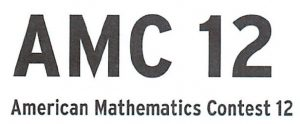AUTHORS

Justin Stevens: Accelerated Math Program Coordinator
University of Alberta – jstevens@cybermath.academy – (909) 713-4398

Forest Kobayashi: Curriculum Designer, Harvey Mudd College

Alex Toller: Curriculum Designer

Advanced High School Math with AMC 10/12 Problems – Mastery Level

Learning objectives

Upon completing this course, you will:
• Have complete mastery of concepts covered in standard Pre-Algebra, Algebra I and Geometry courses, as well as topics not covered in traditional school curriculum.
• Be able to explain and employ important theorems and techniques used in Combinatorics, Number Theory, and Geometry.
• Be able to reduce unfamiliar problems to basic principles, and cleverly employ techniques they’ve learned to find shortcuts in solution methods.

This class emphasizes concepts in mathematics that are more versatile and apply to other facets of your intellectual pursuits. The following is a more comprehensive list of goals that would serve you well in not just math courses, but the field of mathematics:

• Be able to construct geometric figures and diagrams from the ground up (rigorously, not using approximations or “it looks close enough”).
• Relate concepts and ideas to other areas of math, or even make inter-disciplinary connections.
• Associate specific queries and commands in problems with certain formulas and their applications.
• Re-derive generalizations of theorems, or entirely different theorems, from the principal ideas of a proof.
• Exercise the appropriate level of mathematical rigor in solutions, and especially in formal proofs. This entails the proper use of theorems, lemmas, and corollaries in a proof, and proving each step of a proof that, in any sense of the word, is not trivial. Essentially, you should be able to recognize the key differences between intuition (implicit knowledge) and mathematically rigorous proof.

Essential topics

• Mathematical proof!
– What is a proof? And why is it important?
– Proof methods (Direct, contradiction, induction (strong, engineer’s), contrapositive, non-constructive, others)
– Examples of false proofs
– Lots and lots of proof-writing practice!

• Algebra:
– Sequences, series, and convergence
– Pascal’s triangle + binomial theorem
– Complex numbers
– Advanced inequalities: AM-GM-HM-QM, Cauchy, etc.
– Substitutions and the power of the ansatz (reasoned guess)
– Finite/forward differences and Lagrange polynomial interpolation – Generating functions
– Cyclotomic polynomials

• Discrete math:

– Logic
∗ Propositional logic and rules
∗ Types of statements
∗ Statements vs. non-statements

– Set theory
∗ Introduction to sets (terminology, definitions)
∗ Power sets
∗ Relations and functions
∗ Peano’s postulates as a framework for R
∗ Injections, surjections, bijections
∗ Cardinal and ordinal numbers
∗ All infinities are not created equal

– Number theory
∗ Properties of primes
∗ Studying the prime distribution
∗ Bases and modular arithmetic
∗ The interplay of GCD and LCM (and B ́ezout’s Theorem)
∗ Fermat’s Little Theorem, Wilson’s Theorem (and proofs)
∗ Euler’s (totient) theorem
∗ The Legendre symbol and quadratic (non)residues

– Combinatorics
∗ Basic rules of counting
∗ Over/under-counting
∗ Inclusion-Exclusion Principle
∗ Pigeonhole principle
∗ Combinations, permutations
∗ Combinatorial identities (stars and bars, Pascal’s identity)
∗ Probability. Basic definitions/rules, conditional probability, applications.
∗ Expected value and linearity of expectation

– Statistics
∗ Introduction to terminology
∗ Statistical fallacies
∗ Interpreting data
· Different types of graphs
· Correlation vs. causation
· Standard deviation, variance, and data distributions

– Graph theory (definitions, applications)
∗ Nodes, edges
∗ Identities
∗ Handshake theorem (combo)
∗ The Konigsberg bridge problem and its implications
∗ Trees/forests/binary trees
∗ Combinatorial and real-world applications
∗ Graph enumeration (Burnside’s lemma, partitioning Z)
∗ Cayley’s Theorem

• Geometry:
– Fundamental definitions
– Axioms and postulates
– Triangle focus: congruence and similarity
– Coordinate geometry (and coordbashing tactics)
– 3D geometry (planes, cross-sections, volume formula proofs)
– Inscribed angle theorem
– Angle chasing
– Power of a point
– Overview of trigonometry:
∗ Definitions (sin, cos, tan and their reciprocals/inverses)
∗ Trig identities and their a priori derivations
∗ Applications to algebra (complex numbers, etc.)
– Laws of sines, cosines
– Polar coordinates and complex numbers

AUTHORS

Alex Toller: Curriculum Designer

### Courses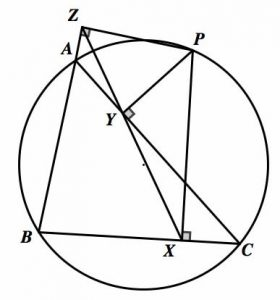To find out what course is best for you, please look at the information below:

There are two tracks in this course:

Advanced AIME with Proofs: For students who can comfortably qualify for the
AIME and solve the first half of problems on the exam. These students might be
aiming to qualify for USA(J)MO and have a pleasant start on the olympiad.

USA(J)MO: For students who can already comfortably qualify for USA(J)MO, and
are aiming to score highly on it.

(1) Starting out on AMC, trying to qualify for AIME

(2) Can solve 2 problems on AIME, hoping to solve 8

(3) Can solve 6+ problems on AIME, hoping to solve 13

(4) Can qualify for USA(J)MO, hoping to solve a problem or two

(5) Can solve one or two USA(J)MO problems and solve hard USAJMO or medium USAMO problems

(6) Aiming to solve the final P3 / P6 problems on USAMO

Our Math Olympiad Program has two tracks:

* Prerequisites: 6+ on AIME

* Aiming for high AIME scores, and a couple problems on USA(J)MO

* Prerequisites: consistently qualify for USA(J)MO

* Aiming to score 14+ on USAMO

Third: Placement

– If you are in levels 1 or 2, you should sign up for our Advanced High School Math with AMC 10/12 Problems course. It covers AMC 10/12 and the non-proof problems on AIME.

– If you are in levels 3 or 4, you should sign up for our Advanced AIME with Proofs course.

– If you are in levels 5 or 6, you should sign up for our USA(J)MO course.

There are two tracks in this course:

Advanced AIME with Proofs: For students who can comfortably qualify for the
AIME and solve the first half of problems on the exam. These students might be
aiming to qualify for USA(J)MO and have a pleasant start on the olympiad.

USA(J)MO: For students who can already comfortably qualify for USA(J)MO, and
are aiming to score highly on it.

These two tracks overlap in any given year. The curriculum runs in a three-year cycle.

Contest preparation: AIME, HMMT, USA(J)MO, IMO.

Curriculum: The course operates on a three-year cycle, so students can repeat the course up to three times total, across both tracks. The summer and year-round materials
are disjoint.

A detailed listing of topics covered appears below. Not all topics occur in all years. Most topics occur in multiple years, but they will have different examples and problems each time they appear over the three-year cycle.

Each iteration of the course contains several practice exams.

1- Topics appearing only in Advanced AIME with Proofs

Algebra

• Symmetric Polynomials. Vieta’s formulas, Newton sums, fundamental theorem of elementary symmetric polynomials.
• Logarithms.Computational problems and equations involving logarithms.
• Trig Equation. Algebraic problems involving trig functions.
• Intro Functional Equation. Introduction to olympiad-style functional equations. Substitutions, injectivity and surjectivity, Cauchy’s functional equation.
• Inequalities. Introduction to olympiad-style inequalities. AM-GM and Cauchy-Schwarz.

Combinatorics

• Computations with Probability. Random variables, expected value, linearity of expectation.
• Enumeration. Computational counting problems.
• Monovariants and Invariants. Finite processes.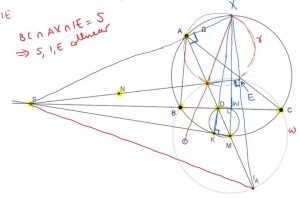Geometry

• Computational Geometry. AIME-style problems in Euclidean geometry.
 Angle Chasing.
• Trig in Geometry.
• Elementary Geometry. Angle chasing, power of a point, homothety.
• Basics of Complex Numbers. Introduction to complex numbers in geometry.

Number theory

• Computations with Modular Arithmetic. Fermat, Wilson, Chinese Remainder theorem.
• Diophantine Equations. Introduction to olympiad-style Diophantine equations.
• Chinese Remainder Theorem.

2- Topics appearing in both tracks

Algebra

• Generating Functions. Their uses in combinatorial sums.
• Linear Recursions and Finite Differences.
• Sums. Swapping order of summation.
• Polynomials. Fundamental theorem of algebra, factorizations, roots.

Combinatorics

• Weights and Colorings.
• Induction and Recursion.
• Linearity of Expectation and Double-Counting.
• Algorithms. Combinatorial problems involving discrete-time processes.
• Graph Theory. Definitions and problems.
• Problems on Rectangular Grids.

Geometry

• Power of a Point.
• Homothety
• Common Congurations.

Number theory

• Divisibility and Euclidean Algorithm. Bounding the remainders.
• Look at the Exponent. p-adic evaluation, lifting the exponent.
• Orders. Primes of the form a2 + b2. Primitive roots.

3- Topics appearing only in USA(J)MO

Algebra

• Functional Equations. More difficult functional equations at the USAMO/IMO level.
• Advanced Inequalities. Jensen and Schur. Fudging, smoothing.
• Analysis and Calculus. Understanding the complete theorem statements in calculus and how they can be applied to olympiad problems. Differentiation and the relation
to multiplicity of roots. Lagrange multipliers. Compactness.

Combinatorics

• Games and Processes.

Geometry

• Projective Geometry. Harmonic bundles, poles and polars.
• Inversion.
• Spiral Similarity.
• Complex Numbers. Applications to problems.
• Barycentric Coordinates. Applications to problems.

Number theory

• Constructions in Number Theory.
• Integer Polynomials. Irreducibility, minimal polynomials, a taste of Galois theory.